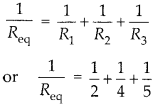Enlightened

# Question 4: NCERT Solutions for 12th Class Physics: Chapter 3-Current Electricity

• 0

Question 4: NCERT Solutions for 12th Class Physics: Chapter 3-Current Electricity

(a) Three resistors 2Ω, 4Ω and 5Ω are combined in parallel. What is the total resistance of the combination?
(b) If the combination is connected to a battery of emf 20 V and negligible internal resistance. Determine the current through each resistor, and the total current drawn from the battery.

Share

1. Solution:
(a) Total resistance of the combination in parallel(b) Potential of 20 V will be same across each resistor, so currentCheck the complete chapter with solutions.

NCERT Solutions for 12th Class Physics: Chapter 3-Current Electricity

• 0